Next: Tidal Bores Up: Incompressible Inviscid Flow Previous: Flow over Shallow Bump

# Stationary Hydraulic Jumps

Under certain circumstances, water flowing within a horizontal open channel (i.e., a stream) of constant width is found to have a depth that changes very rapidly over a short section of the channel. This phenomenon is known as a hydraulic jump, and is illustrated in Figure 4.6.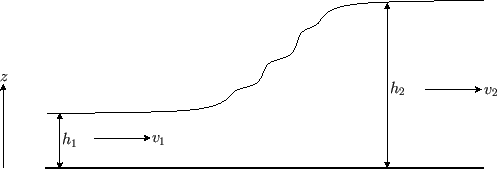Suppose that the jump is stationary. Let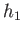,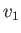, and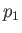be the depth, flow velocity, and pressure, of the water, respectively, upstream of the jump. Similarly let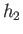,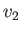, and, be the depth, flow velocity and pressure, respectively, downstream of the jump. As before,andare assumed to be uniform across the channel. We are also assuming that the flow is laminar (i.e., smooth) both upstream and downstream of the jump. Note that the region of the channel in which the jump occurs is generally associated with violent mixing that gives rise to significant transfer of mechanical energy from the laminar (i.e., smooth) to the turbulent component of the flow. (The latter component is localized in the vicinity of the jump, and is continuously dissipated by viscosity on small-scales.) Consequently, we cannot use Bernoulli's equation to analyze the jump, because this equation assumes that the laminar component of the flow conserves mechanical energy. However, we can still make use of fluid continuity, as well as the Euler momentum theorem, neither of which depend on the conservation of mechanical energy.

Fluid continuity yields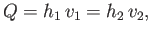(4.37)

where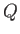is the fixed flow rate per unit width. Furthermore, the Euler momentum theorem (see Section 4.4) implies that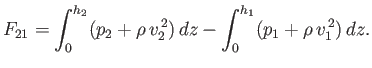(4.38)

Here,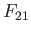is the horizontal thrust per unit width exerted by the channel bed on the water lying between pointsand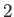. Moreover,measured vertical height above the bed. Assuming the usual linear pressure variation with depth, we can write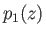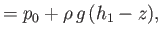(4.39)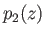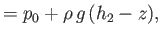(4.40)

where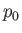is atmospheric pressure. Hence, we deduce that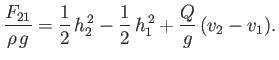(4.41)

The neglect of frictional drag at the channel bed implies that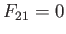. Thus, we obtain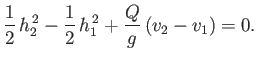(4.42)

The previous equation possesses the trivial solution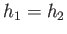and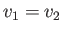, which corresponds to the absence of a hydraulic jump. Eliminatingandbetween Equations (4.37) and (4.42), and canceling a common factor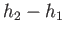, we obtain the non-trivial solution(4.43)

Now, the upstream Froude number is defined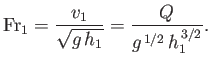(4.44)

Eliminatingbetween the previous two equations, we obtain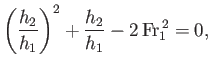(4.45)

which can be solved to give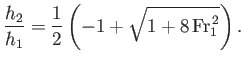(4.46)

Here, we have neglected an unphysical solution in which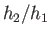is negative. Note that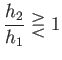as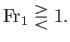(4.47)

The downstream Froude number is defined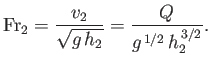(4.48)

Eliminatingbetween Equations (4.43) and (4.48), we obtain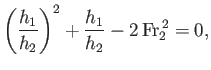(4.49)

which can be solved to give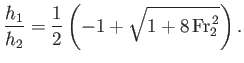(4.50)

Here, we have again neglected an unphysical solution in which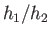is negative. We can combine Equations (4.46) and (4.50) to give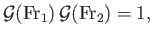(4.51)

where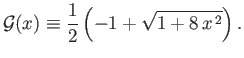(4.52)

Note that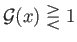as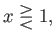(4.53)

which implies that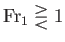as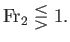(4.54)

In other words, if the upstream flow is super-critical then the downstream flow is sub-critical, and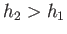. On the other hand, if the upstream flow is sub-critical then the downstream flow is super-critical, and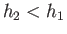.

By analogy with Equation (4.18), we can define the head heights of the flows upstream and downstream of the jump as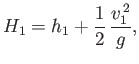(4.55)

and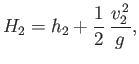(4.56)

respectively. Of course, in the absence of any transfer of mechanical energy from the laminar to the turbulent component of the flow, within the jump, we would expect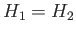. (Because this is what Bernoulli's equation predicts.) In the presence of the transfer, we expect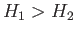. In other words, we expect there to be a head loss across the jump. Note that it is impossible for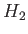to exceed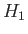, because this would imply a transfer of mechanical energy from the turbulent to the laminar component of the flow, within the jump, which violates the second law of thermodynamics. Let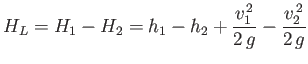(4.57)

be the positive definite head loss. Making use of some previous definitions, we can write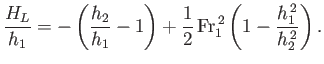(4.58)

Now, according to Equation (4.45),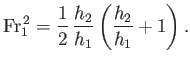(4.59)

Hence, combining the previous two equations, we obtain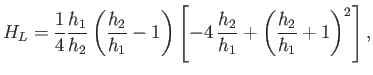(4.60)

or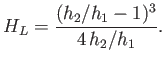(4.61)

Thus, the thermodynamic constraint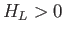implies that. However, as we have already seen,implies that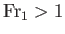. In other words, a stationary hydraulic jump can only occur when the upstream flow is super-critical, and the downstream flow sub-critical.

A hydraulic jump often occurs at the base of a spillway from a dam, where the flow is accelerated to super-critical speeds. Because a hydraulic jump always results in a loss of mechanical energy from the flow, spillways are sometimes designed to promote jumps, so as to deliberately remove energy from the flow, thereby reducing the danger from excessive currents in flood control.Next: Tidal Bores Up: Incompressible Inviscid Flow Previous: Flow over Shallow Bump
Richard Fitzpatrick 2016-03-31